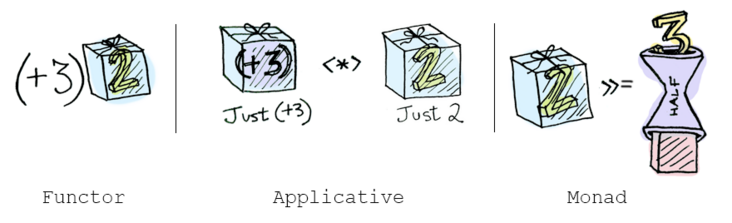0收藏Promise 也是一种 Functor ?

Javascript Array 的 map 方法，相信开发者们都很熟悉，并且几乎在每天的编程中都会用到，但 Array 原型链上的另一个方法 Array.prototype.flatMap 可能很多人没怎么用过，从字面意思上理解就是扁平化的 map，实际作用也确实是的，看一个和 map 做对比的使用案例：

``````const arr = [1, 2, 3, 4]
arr.map(x => [x * 2])  // => [, , , ]
arr.flatMap(x => [x * 2])  // => [2, 4, 6, 8]``````

flatMap 相对于 map 的区别是：

• map 是把函数执行的结果，放在一起然后装进 Box 中；
• flatMap 的结果是把函数执行的结果分别去掉一层“包装”，然后放在一起装进 Box 中

``````const compose = (...fns) => x => fns.reduceRight((v, f) => f(v), x)
const user = {
street: '长河'
}
}
const res = app(user) // => 长河``````

``````const address = user => fromNullable(user).map(u => u.address)
const app = user =>
.map(street)  // Either(Either(street))
const res = app(user) // => Rirgt(Right('长河'))``````

``````const app = user =>
.map(s => street(s).fold(x => x, x => x))   // Either(street)
.fold(() => 'default street', x => x)       // street

const res = app(user)  // => '长河'``````出现两层包装的原因是：map 会把函数计算的结果重新包装进 Box 中，而这一层包装有点赘余，因为之后立即进行了拆箱操作，这非常类似于 Array flatmap (先 map 然后 flat )。

``````const Right = x => ({
flatMap: f => f(x),
});
const Left = x => ({
flatMap: f => Left(x),
});
const app = user =>
.flatMap(street)                      // Either(street)
.flod(() => 'default street',x => x)  // street``````

map 和 flatMap 的不同点：map 方法接收一个仅仅变换容器内值的函数，所以需要用 Box 重新包装；但是 flatMap 接收一个返回Box类型的函数，直接调用即可。
map 和 flatMap 的相同点却是非常明显的：都是返回一个 Box 的实例，方便后面继续链式的调用。

flatMap 方法和 flod 方法逻辑一样？这里得承认他们确实很类似，但是他们的使用场景却完全不同！flod 的用途是把一个值从 Box 中解放出来；flatMap 的用途是把一个返回 Box 的函数应用到一个 Box 上，这样后面可以继续保持链式的调用。

``````const readConfig = (filepath) => {
try {
const config = JSON.parse(str);
return config.version
} catch (e) {
return '0.0.0'
}
}
console.log(result) // => '1.0.0'``````

``````const readConfig = (filepath) =>
.chain(json => tryCatch(() => JSON.parse(json)))  // Either('')
.fold(() => '0.0.0', c => c.version)
console.log(result) // => '1.0.0'``````

“A monad is just a monoid in the category of endofunctors. What’s the problem?”All told, a monad in X is just a monoid in the category of endofunctors of X, with product × replaced by composition of endofunctors and unit set by the identity endofunctor. -- Saunders Mac Lane

callback 与 异步

``````const getUrl = url => callback => request(url, callback)
const getSomeJSON = getUrl('http://example.com/somedata.json')
getSomeJSON((err, data)=>{
if(err){
//do something with err
}else {
//do something with data
}
})``````

1.函数的调用者无法直接控制请求，必须要把所有的后续操作放在 callback 里面
2.函数无法组合，因为 getSomeJSON 调用之后没有返回任何结果

``````const getUrl = url =>
(reject, resolve) =>
request(url, (err, data)=>{
err? reject(err) : resolve(data)
})``````

``````const getSomeJSON = getUrl('http://example.com/somedata.json')
const handleError = err => console.error('Error fetching JSON', err)
const handleData = compose(renderData, JSON.parse)
getSomeJSON(handleError, handleData) // 触发请求``````

``````const stringifyJson= '{"a":1}'
LazyBox(() => stringifyJson).map(JSON.parse) // => LazyBox({ a: 1 })``````

LazyBox 用来包裹同步的函数，那么同理对于处理异步逻辑的 fork 函数，也可以用一个盒子包装起来，然后 map 普通函数 f ，不也可以实现函数组合吗？对于异步的逻辑，可以称之为 Task (任务：将来才会完成某个目标或者达成某种结果，是不是很好理解)

``````const Task = fork => ({
map: f => Task((reject, resolve) =>          // return another Task, including a new fork.
fork(reject, x => resolve(f(x)))),   // when called,the new fork will run `f` over the value, before calling `resolve`
fork,
})``````

Task 的 map 方法，接收一个函数 f ，返回一个新的 Task，关键点在：新的 fork 函数会调用上一个 fork ，如果是正确的分支则 resolve 被函数 f 计算过后的结果，如果是失败的分支，则传递 reject。

``````const readConfig = filepath => Task((reject, resolve) =>
err ? reject(err) : resolve(data)
))
.map(JSON.parse)
app.fork(() => console.log('something went wrong'), json => console.log('json', json))``````

Task.map 和 LazyBox 的 map 完全类似，一直都是在做函数组合的工作，并没有进行实际的函数调用，LazyBox 最后通过调用 fold 真正实现函数调用，而 Task 最后通过调用 fork ，实现异步函数的执行。

``````const app = readConfig(readPath)
.map(JSON.parse)
.map(c => ({ version: c.version + 1 }))
.map(JSON.stringify)
const writeConfig = (filepath, contents) =>
fs.writeFile(filepath, contents, (err, _) =>
err ? reject(err) : resolve(contents)
)
})``````

``````const app = readConfig(readPath)
.map(JSON.parse)
.map(c => ({ version: c.version + 1 }))
.map(JSON.stringify)
.chain(c => writeConfig(writeFilepath, c))
app.fork(() => console.log('something went wrong'),
() => console.log('read and write config success'))``````

``````const httpGet = content => Task((rej, res) => {
setTimeout(() => res(content), 2000)
})
const getUser = (id) => httpGet('Melo')
const getAge = name => httpGet(name)
getUser('id')
.chain(name => getAge(name + ' 18'))
.fork(console.error, console.log) // => 4000ms later， log： "Melo 18"``````

``````const readConfig = filepath => new Promise((resolve, reject) =>
err ? reject(err) : resolve(data)
))
const writeConfig = (filepath, contents) => new Promise((resolve, reject) => {
fs.writeFile(filepath, contents, (err, _) =>
err ? reject(err) : resolve(contents)
)
})
.then(JSON.parse)
.then(c => ({ version: c.version + 1 }))
.then(JSON.stringify)
.then(c => writeConfig(writeFilepath, c))``````

``````const Box = x => ({
map: f => Box(f(x)),
chain: f => f(x),
})
const box1 = Box(1)                          // => Box(1)
const promise1 = Promise.resolve(1)          // => Promise(1)

box1.map(x => x + 1)                         // => Box(2)
promise1.then(x => x + 1)                    // => Promise(2)
// -----------------
box1.chain(x => Box(x + 1))                 // => Box(2)
promise1.then(x => Promise.resolve(x + 1))  // => Promise(2)``````

``````box1.map(x => Box(x + 1))                   // => Box(Box(2))
promise1.then(x => Promise.resolve(x + 1))  // => Promise(2)
box1.chain(x => x + 1)                      // => 2
promise1.then(x => x + 1)                   // => Promise(2)``````

Monad 更擅长处理的是一种拥有 Context(上下文) 的场景，上面的 getUser 与 getAge 的例子中，getAge 函数必须等到 getUser 函数中的异步执行完成才能开始调用，这是一种纵向(串行)的链路；

``````Task
.of(name => age => ({ name, age }))
.ap(getUser)
.ap(getAge)
.fork(console.error, console.log) // 2000ms later， log： "{name: 'Melo', age: 18}"``````

• Functor 是一种实现 map 方法的数据类型
• Applicative 是一种实现了 apply 方法的数据类型
• Monad 是一种实现了 chain 或 flatmap 方法的数据类型• Functor: 应用一个函数到包裹的值，使用 map.
• Applicative: 应用一个包裹的函数到包裹的值，使用 ap

Array.prototype.flatMap
Understanding Functor and Monad With a Bag of Peanuts
Functors, Applicatives, And Monads In Pictures
A Brief, Incomplete, and Mostly Wrong History of Programming Languages
The Little Idea of Functional Programming
Comparison to Promises
Functional Programming In JavaScript — With Practical Examples
Translation from Haskell to JavaScript of selected portions of the best introduction to monads I’ve ever read

《JavaScript函数式编程》帖子
视频
声望
粉丝
最近发布
相关问题
社区精华内容